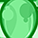# MOSFET (Si/SiC) Forum DiscussionsLevel 1
Level 1# IRLML2803 leakage current

Hi,

I am using your part IRLML2803 in my design and need to understand how the drain to source leakage current varies with temperature.

The part datasheet states the leakage current for 25°C and 125°C. I would like to understand how to extrapolate the leakage at a temperature of 100°C?

In addition can you provide some information on how the worst case value is selected over the nominal value, is a statistical method used to provide this figure?

Many thanks

Simon

1 SolutionModeratorModerator# Re: IRLML2803 leakage current

Hello @Simon_C ,

Drain to source leakage current is characterized at certain Tj values. The Idss variation with respect to temperature is exponential. In practice, an equation is derived through exponential regression using which, the Idss at different intermediate Tj could be derived.

As a rule of thumb, we can see 10 times increase in Idss for increase of every 50°C.

Characterization is done with using certain standard sample set. And the nominal value is the mean value of this measured dataset, while worst case value is defined based on the max value from the measurement plus a safety margin.

Can you please let us know the application for which the device is being considered?

Regards

MeghanaModeratorModerator# Re: IRLML2803 leakage current

Hello @Simon_C ,

Drain to source leakage current is characterized at certain Tj values. The Idss variation with respect to temperature is exponential. In practice, an equation is derived through exponential regression using which, the Idss at different intermediate Tj could be derived.

As a rule of thumb, we can see 10 times increase in Idss for increase of every 50°C.

Characterization is done with using certain standard sample set. And the nominal value is the mean value of this measured dataset, while worst case value is defined based on the max value from the measurement plus a safety margin.

Can you please let us know the application for which the device is being considered?

Regards

Meghana